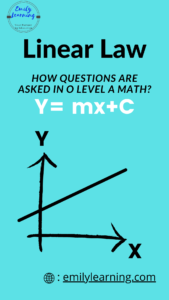# Tag: AMath Linear Law## Linear Law A Math Questions are Predictable

Linear Law questions in A Math are very predictable! Because questions from this topic are predictable, it is easy to score for Linear Law O…## Summary Notes for Linear Law – O Level Add Math

Linear law is a chapter that revolves around making a non-linear relationship linear by changing our concept of y must be the vertical axis and…## What is tested for linear law tested in O Level Additional Mathematics

Let’s talk about what you need to know about linear law in O Level Additional Mathematics. Basically, in this topic, you are required to convert…

error: Content is protected !!